# NMath User's Guide

21.1 Ordinary Least Squares Methods (.NET, C#, CSharp, VB, Visual Basic, F#)

NMath includes least squares classes that compute solutions using various methods: Cholesky factorization, QR decomposition, and singular value decomposition. The interface is virtually identical for all least squares classes.

Least Squares Using Cholesky Factorization

The Cholesky least squares classes solve least square problems by using the Cholesky factorization to solve the normal equations. The normal equations for the least squares problem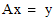are: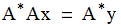where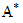denotes the transpose of a real matrix A or the conjugate transpose of a complex matrix A. If A has full rank, then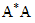is symmetric positive definite­­—the converse is also true—and the Cholesky factorization may be used to solve the normal equations. This method will fail if the matrix A is rank deficient.

Finding least squares solutions using the normal equations is often the best method when speed is the only consideration.

Least Squares Using QR Decomposition

The QR decomposition least squares classes solve least squares problems by using a QR decomposition to find the minimal norm solution to the linear system. That is, they find the vector x that minimizes the 2-norm of the residual vector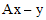. Matrix A must have more rows than columns, and be of full rank.

Finding least squares solutions via QR decomposition is the "standard" method for least squares problems, and is recommended for general use.

Least Squares Using SVD

If the matrix A is close to rank-deficient, the QR decomposition method described above has less than ideal stability properties. In such cases, a method based on singular value decomposition is a better choice.

Top

Top